# Elementary Algebra Textbook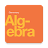4.7
46 reviews
10K+Everyone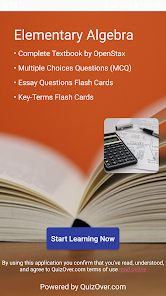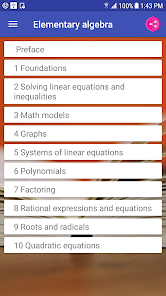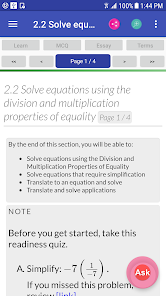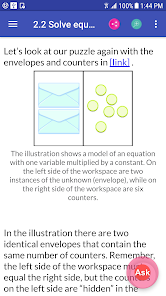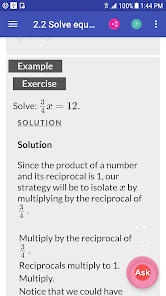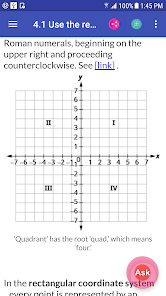Elementary algebra

* Complete Textbook by OpenStax
* Multiple Choices Questions (MCQ)
* Essay Questions Flash Cards
* Key-Terms Flash Cards

1. Foundations
1.1. Introduction to whole numbers
1.2. Use the language of algebra
1.4. Multiply and divide integers
1.5. Visualize fractions
1.7. Decimals
1.8. The real numbers
1.9. Properties of real numbers
1.10. Systems of measurement
2. Solving linear equations and inequalities
2.1. Solve equations using the subtraction and addition properties of equality
2.2. Solve equations using the division and multiplication properties of equality
2.3. Solve equations with variables and constants on both sides
2.4. Use a general strategy to solve linear equations
2.5. Solve equations with fractions or decimals
2.6. Solve a formula for a specific variable
2.7. Solve linear inequalities
3. Math models
3.1. Use a problem-solving strategy
3.2. Solve percent applications
3.3. Solve mixture applications
3.4. Solve geometry applications: triangles, rectangles, and the pythagorean theorem
3.5. Solve uniform motion applications
3.6. Solve applications with linear inequalities
4. Graphs
4.1. Use the rectangular coordinate system
4.2. Graph linear equations in two variables
4.3. Graph with intercepts
4.4. Understand slope of a line
4.5. Use the slope–intercept form of an equation of a line
4.6. Find the equation of a line
4.7. Graphs of linear inequalities
5. Systems of linear equations
5.1. Solve systems of equations by graphing
5.2. Solve systems of equations by substitution
5.3. Solve systems of equations by elimination
5.4. Solve applications with systems of equations
5.5. Solve mixture applications with systems of equations
5.6. Graphing systems of linear inequalities
6. Polynomials
6.2. Use multiplication properties of exponents
6.3. Multiply polynomials
6.4. Special products
6.5. Divide monomials
6.6. Divide polynomials
6.7. Integer exponents and scientific notation
7. Factoring
7.1. Greatest common factor and factor by grouping
7.4. Factor special products
7.5. General strategy for factoring polynomials
8. Rational expressions and equations
8.1. Simplify rational expressions
8.2. Multiply and divide rational expressions
8.3. Add and subtract rational expressions with a common denominator
8.4. Add and subtract rational expressions with unlike denominators
8.5. Simplify complex rational expressions
8.6. Solve rational equations
8.7. Solve proportion and similar figure applications
8.8. Solve uniform motion and work applications
8.9. Use direct and inverse variation
9.1. Simplify and use square roots
9.2. Simplify square roots
9.3. Add and subtract square roots
9.4. Multiply square roots
9.5. Divide square roots
9.6. Solve equations with square roots
9.7. Higher roots
9.8. Rational exponents
Updated on
Mar 20, 2018

## Data safety

Safety starts with understanding how developers collect and share your data. Data privacy and security practices may vary based on your use, region, and age. The developer provided this information and may update it over time.No data shared with third partiesNo data collected
4.7
46 reviews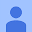January 28, 2020
Great!!! This app helped me to learn algebra from zero. Although, it would be perfect if the links worked. Aunque hablo español el lenguaje es sencillo y fácil de entender con nivel intermedio. I thank developers for the effort they have made creating this app.
15 people found this review helpful